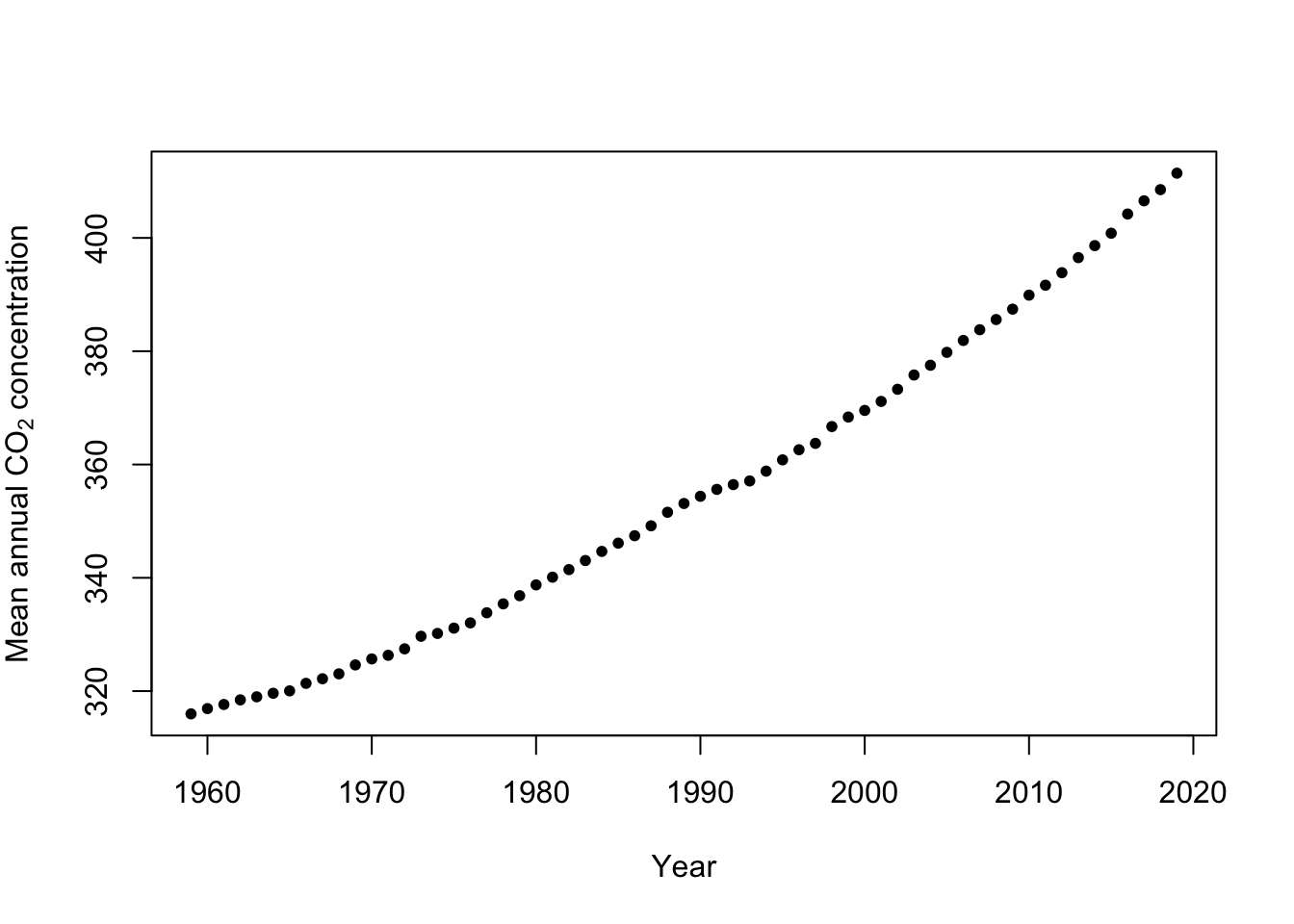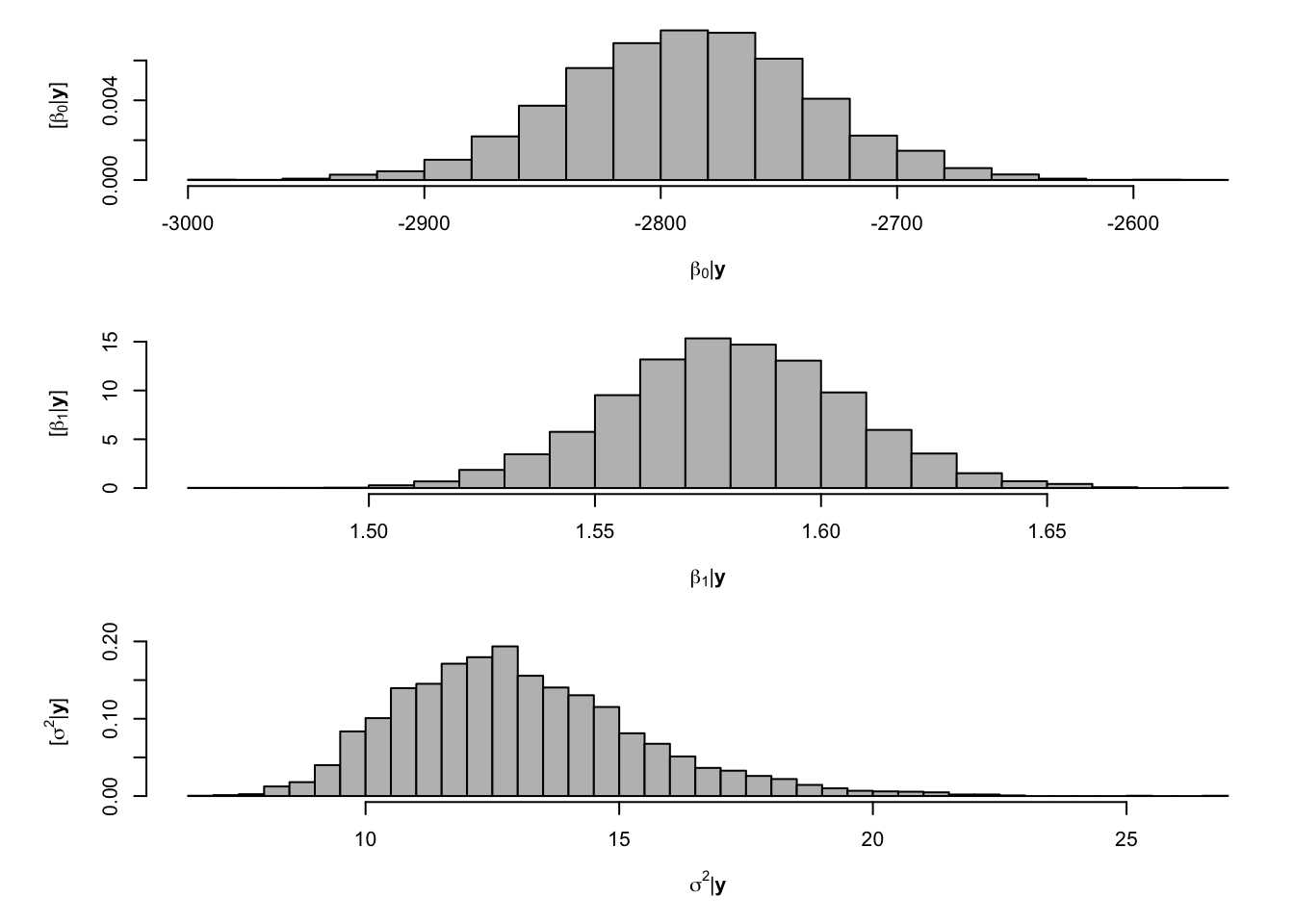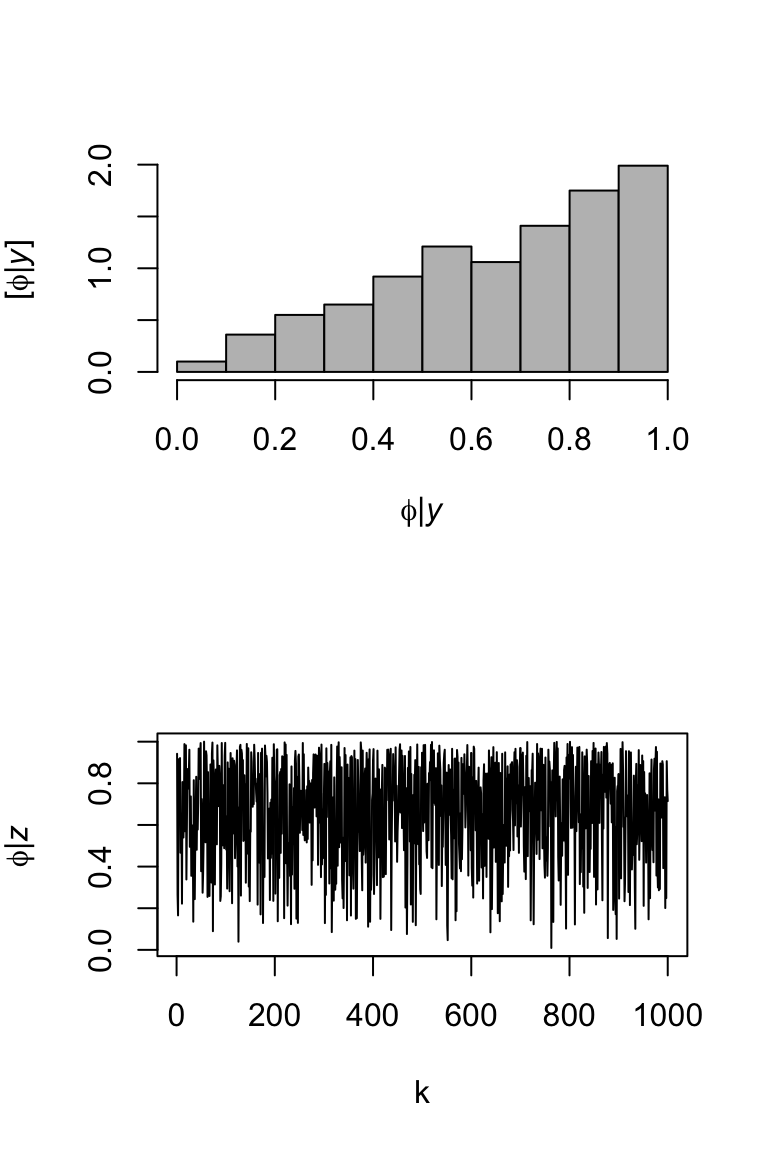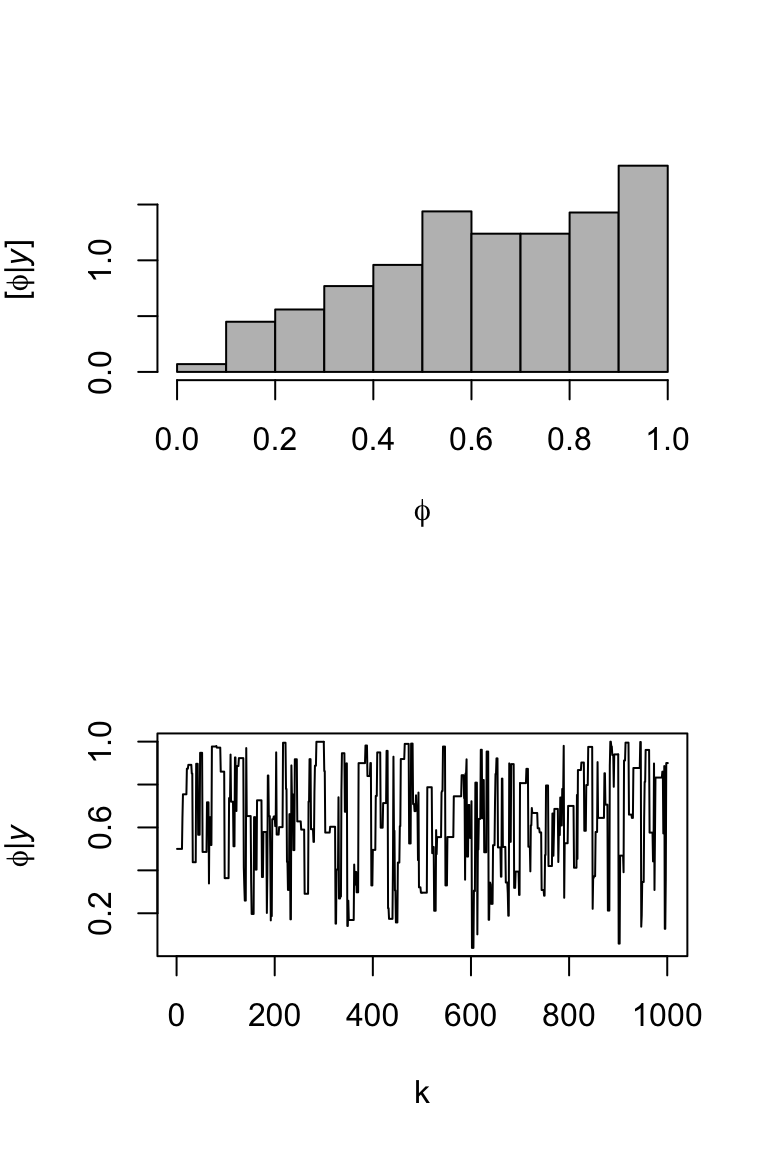# 11 September 22

## 11.1 Announcements

• Assignment #3 is posted and due next week.

## 11.2 Extreme precipitation in Kansas

• What we will need to learn
• How to use R as a geographic information system
• New general tools from statistics
• Gaussian process
• Metropolis and Metropolis–Hastings algorithms
• Gibbs sampler
• How to use the hierarchical modeling framework to describe Kriging
• Hierarchical Bayesian model vs. “empirical” hierarchical model
• Specialized language used in spatial statistics (e.g., range, nugget, variogram)

## 11.3 Gibbs sampler

• History
• Stochastic Relaxation, Gibbs Distributions, and the Bayesian Restoration of Images (Geman and Geman 1984))
• Sampling-Based Approaches to Calculating Marginal Densities (Gelfand and Smith 1990)
• The gibbs sampler is responsible for the recent “Bayesian revolution”
• Gibbs sampler
• See pg. 169 in book.
• In many cases the distribution we want to sample from is multivariate
• If we can sample from the univariate full-conditional distribution for each random variable of a multivariate distribution, then we can use a Gibbs sampler to obtain dependent sample from the multivariate distribution

### 11.3.1 Gibbs sampler: simple example

$\boldsymbol{\theta}\sim\text{N}\Bigg(\mathbf{0},\bigg[\begin{array}{cc} 1 & \rho\\ \rho\ & 1 \end{array}\bigg]\Bigg)$ where $$\boldsymbol{\theta}\equiv(\theta_{1},\theta_{2})'$$. Suppose we didn’t know how to sample from the multivariate normal distribution, but we could obtain the conditional distributions $$[\theta_{1}|\theta_{2}]$$ and $$[\theta_{2}|\theta_{1}]$$ which are $\theta_{1}|\theta_{2}\sim\text{N}(\rho\theta_{2},1-\rho^{2})$ $\theta_{2}|\theta_{1}\sim\text{N}(\rho\theta_{1},1-\rho^{2})\,.$ It turns out that if we iteratively sample from $$[\theta_{1}|\theta_{2}]$$ and then $$[\theta_{2}|\theta_{1}]$$ (or the other way around) we will obtain samples from $$[\boldsymbol{\theta}]$$.

### 11.3.2 Gibbs sampler: Bayesian linear model example

• The model
• Distribution of the process: “$$\mathbf{y}$$ given $$\boldsymbol{\beta}$$ and $$\sigma_{\varepsilon}^{2}$$$\mathbf{y}|\boldsymbol{\beta},\sigma_{\varepsilon}^{2}\sim\text{N}(\mathbf{X}\boldsymbol{\beta},\sigma_{\varepsilon}^{2}\mathbf{I})$ This is also sometimes called the process model.
• Distribution of the parameters: $$\boldsymbol{\beta}$$ and $$\sigma_{\varepsilon}^{2}$$ $\boldsymbol{\beta}\sim\text{N}(\mathbf{0},\sigma_{\beta}^{2}\mathbf{I})$ $\sigma_{\varepsilon}^{2}\sim\text{inverse gamma}(q,r)$
• Computations and statistical inference
• Using Bayes rule (Bayes 1763) we can obtain the joint posterior distribution $[\boldsymbol{\beta},\sigma_{\varepsilon}^{2}|\mathbf{y}]=\frac{[\mathbf{y}|\boldsymbol{\beta},\sigma_{\varepsilon}^{2}][\boldsymbol{\beta}][\sigma_{\varepsilon}^{2}]}{\int\int [\mathbf{y}|\boldsymbol{\beta},\sigma_{\varepsilon}^{2}][\boldsymbol{\beta}][\sigma_{\varepsilon}^{2}]d\boldsymbol{\beta}d\sigma_{\varepsilon}^{2}}$
• Statistical inference about a paramters is obtained from the marginal posterior distributions $[\boldsymbol{\beta}|\mathbf{y}]=\int[\boldsymbol{\beta},\sigma_{\varepsilon}^{2}|\mathbf{y}]d\sigma_{\varepsilon}^{2}$ $[\sigma_{\varepsilon}^{2}|\mathbf{y}]=\int[\boldsymbol{\beta},\sigma_{\varepsilon}^{2}|\mathbf{y}]d\boldsymbol{\beta}$
• In practice it is difficult to find closed-form solutions for the marginal posterior distributions, but easy to find closed-form solutions for the conditional posterior distribtuions.
• Full-conditional distribtuions: $$\boldsymbol{\beta}$$ and $$\sigma_{\varepsilon}^{2}$$ $\boldsymbol{\beta}|\sigma_{\varepsilon}^{2},\mathbf{y}\sim\text{N}\bigg{(}\big{(}\mathbf{X}^{\prime}\mathbf{X}+\frac{1}{\sigma_{\beta}^{2}}\mathbf{I}\big{)}^{-1}\mathbf{X}^{\prime}\mathbf{y},\sigma_{\varepsilon}^{2}\big{(}\mathbf{X}^{\prime}\mathbf{X}+\frac{1}{\sigma_{\beta}^{2}}\mathbf{I}\big{)}^{-1}\bigg{)}$ $\sigma_{\varepsilon}^{2}|\mathbf{\boldsymbol{\beta}},\mathbf{y}\sim\text{inverse gamma}\left(q+\frac{n}{2},(r+\frac{1}{2}(\mathbf{y}-\mathbf{X}\boldsymbol{\beta})^{'}(\mathbf{y}-\mathbf{X}\boldsymbol{\beta}))^-1\right)$
• How do you find the full-conditional distributions?
• Gibbs sampler for the Bayesian linear model
• Write out on the whiteboard
• Example: Mauna Loa Observatory carbon dioxide data

url <- "ftp://aftp.cmdl.noaa.gov/products/trends/co2/co2_annmean_mlo.txt"
colnames(df) <- c("year", "meanCO2", "unc")
plot(x = df$year, y = df$meanCO2, xlab = "Year", ylab = expression("Mean annual " *
CO * " concentration"), col = "black", pch = 20)• Gibb sampler
# Preliminary steps
library(mvnfast)  # Required package to sample from multivariate normal distribution

y <- df$meanCO2 # Response variable X <- model.matrix(~year, data = df) # Design matrix n <- length(y) # Number of observations p <- 2 # Dimensions of beta m.draws <- 5000 #Number of MCMC samples to draw samples <- as.data.frame(matrix(, m.draws, 3)) # Samples of the parameters we will save colnames(samples) <- c(colnames(X), "sigma2") sigma2.e <- 1 # Starting values for sigma2 sigma2.beta <- 10^9 #Prior variance for beta q <- 2.01 #Inverse gamma prior with E() = 1/(r*(q-1)) and Var()= 1/(r^2*(q-1)^2*(q-2)) r <- 1 # MCMC algorithm for (m in 1:m.draws) { # Sample beta from full-conditional distribution H <- solve(t(X) %*% X + diag(1/sigma2.beta, p)) h <- t(X) %*% y beta <- t(mvnfast::rmvn(1, H %*% h, sigma2.e * H)) # Sample sigma from full-conditional distribution q.temp <- q + n/2 r.temp <- (1/r + t(y - X %*% beta) %*% (y - X %*% beta)/2)^-1 sigma2.e <- 1/rgamma(1, q.temp, , r.temp) # Save samples of beta and sigma samples[m, ] <- c(beta, sigma2.e) } # Look a histograms of posterior distributions par(mfrow = c(3, 1), mar = c(5, 6, 1, 1)) hist(samples[, 1], xlab = expression(beta * "|" * bold(y)), ylab = expression("[" * beta * "|" * bold(y) * "]"), freq = FALSE, col = "grey", main = "", breaks = 30) hist(samples[, 2], xlab = expression(beta * "|" * bold(y)), ylab = expression("[" * beta * "|" * bold(y) * "]"), freq = FALSE, col = "grey", main = "", breaks = 30) hist(samples[, 3], xlab = expression(sigma^2 * "|" * bold(y)), ylab = expression("[" * sigma^2 * "|" * bold(y) * "]"), freq = FALSE, col = "grey", main = "", breaks = 30)# Mean of the posterior distribution colMeans(samples[, 1:2]) ## (Intercept) year ## -2787.515552 1.580116 # Compare to results obtained from lm function m1 <- lm(y ~ X - 1) coef(m1) ## X(Intercept) Xyear ## -2787.874923 1.580296 ## 11.4 Metropolis algorithm • Metropolis algorithm • Suppose we know the analytical form of a function that is proportional to some density function but we don’t know how to sample from it. • Example: $$[\phi|y]\propto [y|\phi][\phi]$$ • The distribution we want to sample from is called the target distribution • Obtain the analytical form of a function $$f(\cdot)$$ that is proportional to the target distribution. • Let $$\phi^{*}$$ be a single draw from a proposal distribution $$[\phi^{*}]$$ that is symmetric and easy to sample from. For convenience, make sure the proposal has the same support as the target distribution • Algorithm 1. set an initial value for $$\phi^{(1)}$$ 2. while k < m do 3. obtain $$\phi^{*}$$ by sampling from $$[\phi^{*}]$$ 4. calculate $$R = \text{min}\big(1,\frac{f(\phi^{*})}{f(\phi^{(k)})}\big)$$ 5. set $$\phi^{(k+1)}$$ to $$\phi^{*}$$ with probability $$R$$ else $$\phi^{(k)}$$ 6. end while • Efficiency and the proposal distribution • The Metropolis algorithm produces dependent samples from the target distribution. • Since we can pick any symmetric proposal distribution, we should pick one that results in a higher effective sample size to increase efficiency. • We should also keep track of the acceptance rate (i.e., when we keep the proposed value $$\phi^{*}$$. • Example (Using normal proposal) library(latex2exp) # Let's sample from a beta distribution m <- 1000 # Number of samples set.seed(2030) phi.iid <- rbeta(m,2,1) library(coda) effectiveSize(phi.iid) ## var1 ## 1000 par(mfrow=c(2,1)) hist(phi.iid,col="grey",freq=FALSE,main="", xlab= TeX('$\\phi | \\mathit{y}$'), ylab = TeX('$\\lbrack\\phi | \\mathit{y}\\rbrack$')) plot(phi.iid,typ="l",xlab="k",ylab= TeX('$\\phi | \\mathit{z}$'))# Now assume we couldn't use rbeta() phi <- matrix(,m+1,1) # (m+1) x 1 vector to save samples of x in phi[1,] <- 0.5 # Starting value set.seed(1603) for(i in 1:m){ phi.star <- rnorm(1,0.5,1) # Normal proposal distribution if((phi.star > 0) & (phi.star < 1)){ R <- min(1,(dbinom(1,1,phi.star)*dbeta(phi.star,1,1))/(dbinom(1,1,phi[i,1])*dbeta(phi[i,1],1,1))) }else{R <- 0} if(R > runif(1)){phi[i+1,] <- phi.star}else{phi[i+1,] <- phi[i,1]} #Retain phi.star with probability R } effectiveSize(phi) ## var1 ## 128.858 par(mfrow=c(2,1)) hist(phi,col="grey",freq=FALSE,main="", xlab= TeX('$\\phi$'), ylab = TeX('$\\lbrack\\phi | \\mathit{y}\\rbrack$')) plot(phi,typ="l",xlab="k",ylab= TeX('$\\phi | \\mathit{y}\$'))## 11.5 Metropolis-Hastings algorithm

• Understanding the Metropolis-Hastings algorithm (Chib and Greenberg 1995)
• Similar to the Metropolis algorithm, but the proposal distribution does not have to be symmetric.
• Modifications to the Metropolis algorithm
• $$R=\text{min}\big(1,\frac{f(\phi^{*})}{f(\phi^{(k)})}\times\frac{[\phi^{(k)}]}{[\phi^{*}]}\big)$$ where $$[\phi^{(k)}]$$ and $$[\phi^{*}]$$ is the pdf/pmf of the proposal distribution evaluated at $$\phi^{(k)}$$ and $$\phi^{*}$$ respectively